Browse by Topic

# New in the Wolfram Language: Enhanced Derivatives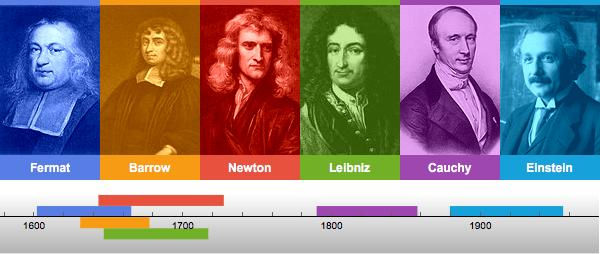Derivatives of functions play a fundamental role in calculus and its applications. In particular, they can be used to study the geometry of curves, solve optimization problems and formulate differential equations that provide mathematical models in areas such as physics, chemistry, biology and finance. The function D computes derivatives of various types in the Wolfram Language and is one of the most-used functions in the system. My aim in writing this post is to introduce you to the exciting new features for D in Version 11.1, starting with a brief history of derivatives.

The idea of a derivative was first used by Pierre de Fermat (1601–1665) and other seventeenth-century mathematicians to solve problems such as finding the tangent to a curve at a point. Given a curve y=f(x), such as the one pictured below, they regarded the tangent line at a point {x,f(x)} as the limiting position of the secant drawn to the point through a nearby point {x,f(x+h)}, as the “infinitesimal” quantity h tends to 0.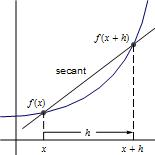Their technique can be illustrated as follows.

The slope of a secant line joining {x,f(x)} and {x+h,f(x+h)} is given by DifferenceQuotient.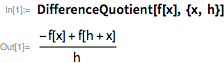Now suppose that the function f(x) is defined as follows.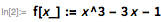Then the slope of a secant line joining {x,f(x)} and {x+h,f(x+h)} is given.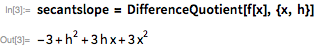The mathematicians of the time then proceeded to find the slope of the tangent by setting h equal to 0.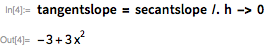The following animation shows the tangent lines along the curve that are obtained by using the formula for the slope derived above.

The direct replacement of the infinitesimal quantity h by 0 works well for simple examples, but it requires considerable ingenuity to compute the limiting value of the difference quotient in more difficult examples. Indeed, Isaac Barrow (1630–1677) and others used geometrical methods to compute this limiting value for a variety of curves. On the other hand, the built-in Limit function in the Wolfram Language incorporates methods based on infinite series expansions and can be used for evaluating the required limits. For example, suppose that we wish to find the derivative of Sin. We first compute the difference quotient of the function.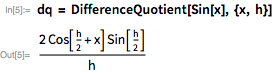Next, we note that setting h equal to 0 directly leads to an Indeterminate expression, as shown below. The Quiet function is used to suppress messages that warn about the indeterminacy.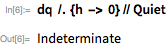Although the direct substitution method has failed, we can use Limit to arrive at the result that the derivative of Sin[x] is Cos[x].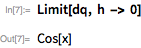Continuing with the historical development, around 1670, Isaac Newton and Gottfried Wilhelm Leibniz “discovered” calculus in the sense that they introduced the general notions of derivative and integral, developed convenient notations for these two operations and established that they are inverses of each other. However, an air of mystery still surrounded the use of infinitesimal quantities in the works of these pioneers. In his 1734 essay The Analyst, Bishop Berkeley called infinitesimals the “ghosts of departed quantities”, and ridiculed the mathematicians of his time by saying that they were “men accustomed rather to compute, than to think.” Meanwhile, calculus continued to provide spectacularly successful models in physics, such as the wave equation for oscillatory motion. These successes spurred mathematicians on to search for a rigorous definition of derivatives using limits, which was finally achieved by Augustin-Louis Cauchy in 1823.

The work of Cauchy and later mathematicians, particularly Karl Weierstrass (1815–1897), laid to rest the controversy about the foundations of calculus. Mathematicians could now treat derivatives in a purely algebraic way without feeling concerned about the treacherous computation of limits. To be more precise, the calculus of derivatives could now be reduced to two sets of rules—one for computing derivatives of individual functions such as Sin, and another for finding derivatives of sums, products, compositions, etc. of these functions. It is this algebraic approach to derivatives that is implemented in D and allows us to directly calculate the derivative of Sin with a single line of input, as shown here.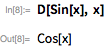Starting from the derivative of a function, one can compute derivatives of higher orders to gain further insight into the physical phenomenon described by the function. For example, suppose that the position s(t) of a particle moving along a straight line at time t is defined as follows.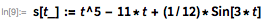Then, the velocity and the acceleration of the particle are given by its first and second derivatives, respectively. The higher derivatives too can be computed easily using D; they also have special names, which can be seen in the following computation.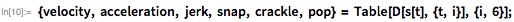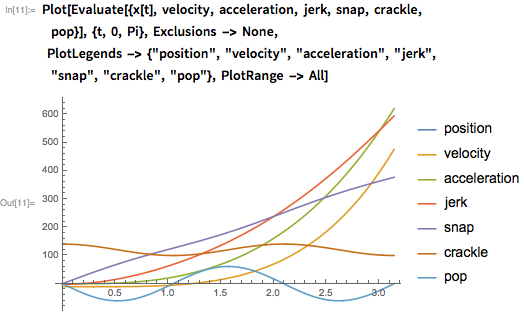Let us now return to our original example and compute the first four derivatives of Sin.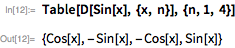There is a clear pattern in the table, namely that each derivative may be obtained by adding a multiple of 𝜋/2 to x, as shown here.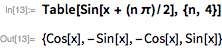In Version 11.1, D returns exactly this formula for the nth derivative of Sin.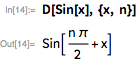An immediate application of the above closed form would be to compute higher-order derivatives of functions with blinding speed. D itself uses this method to compute the billionth derivative of Sin in a flash, using Version 11.1.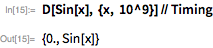The Wolfram Language has a rich variety of mathematical functions, starting from elementary functions such as Power to advanced special functions such as EllipticE. The nth derivatives for many of these functions can be computed in closed form using D in Version 11.1. The following table captures the beauty and complexity of these formulas, each of which encodes all the information required to compute higher derivatives of a given function.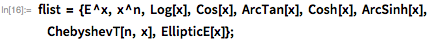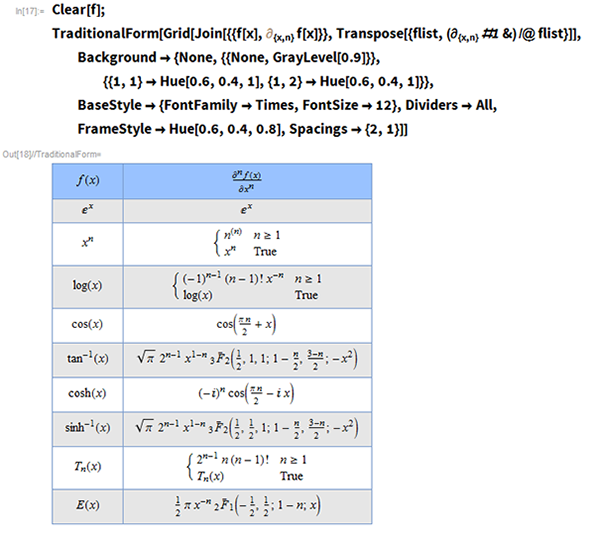Some of the entries in the table are rather simple. For example, the first entry states that all the derivatives of the exponential function are equal to the function itself, which generalizes the following result from basic calculus.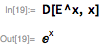In sharp contrast to that, the nth derivative for ArcTan is given by a formidable expression involving HypergeometricPFQRegularized.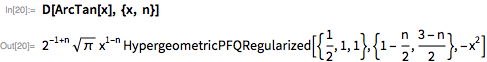If we now give specific values to n in that formula, we obtain elementary answers from the first few derivatives.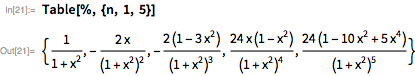These answers agree with the ones obtained if D is used separately for each derivative computation. The results are then simplified.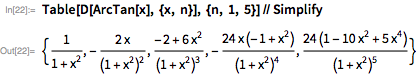The familiar sum, product and chain rules of calculus generalize very nicely to the case of nth derivatives. The sum rule is the easiest, and simply states that the nth derivative of a sum is the sum of the nth derivatives.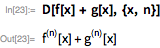The product rule, or the so-called Leibniz rule, gives an answer that is essentially a binomial expansion, expressed as a sum wrapped in Inactive to prevent evaluation.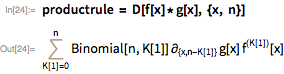We can recover the product rule from a first course on derivatives simply by setting n=1 and applying Activate to evaluate the resulting inert expression.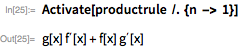Finally, there is a form of the chain rule due to the pious Italian priest Francesco Faà di Bruno (1825–1888). This is given by a rather messy expression in terms of BellY, and states that: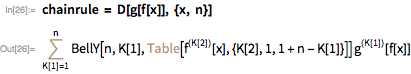Once again, it is easy to recover the chain rule for first derivatives by setting n=1 as we did earlier.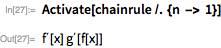The special functions in the Wolfram Language typically occur in families, with different members of each family labeled by integers or other parameters. For example, there is one function BesselJ[n,z] for each integer n. The first four members of this family are pictured below (the sinusoidal character of Bessel functions helps in the modelling of circular membranes).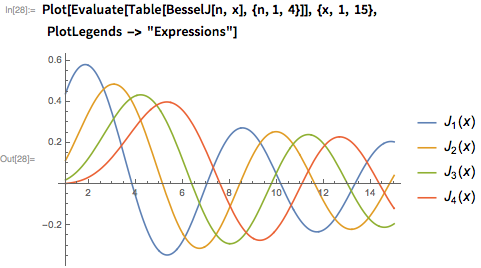It turns out that the derivatives of BesselJ[n,z] can be expressed in terms of other Bessel functions from the same family. While earlier versions did make some use of these relationships, Version 11.1 exploits them more fully to return compact answers for examples such as the following, which generated 210=1024 instances of BesselJ in earlier releases!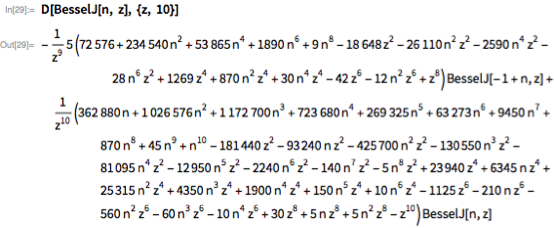The functions considered so far are differentiable in the sense that they have derivatives for all values of the variable. The absolute value function provides a standard example of a non-differentiable function, since it does not have a derivative at the origin. Unfortunately, the built-in Abs function is defined for complex values, and hence does not have a derivative at any point. Version 11.1 overcomes this limitation by introducing RealAbs, which agrees with Abs for real values, as seen in the following plot.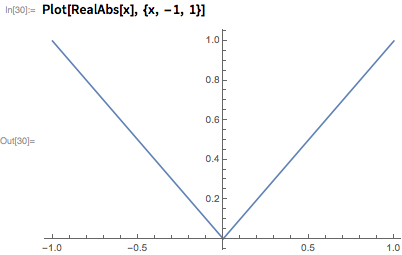This function has a derivative at all values except at the origin, which is given by: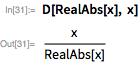The introduction of RealAbs is sure to be welcomed by users who have long requested such a function for use in differential equations and other applications.

This real absolute value function is continuous and only mildly non-differentiable, but in 1872, Karl Weierstrass stunned the mathematical world by introducing a fractal function that is continuous at every point but differentiable nowhere. Version 11.1 introduces several fractal curves of this type, which are named after their discoverers. Approximations for a few of these curves are pictured here.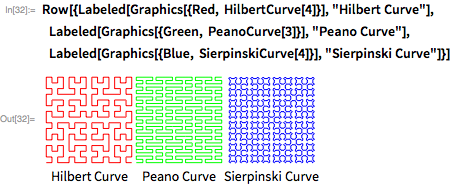Albert Einstein’s 1916 paper announcing the general theory of relativity provided a great impetus to the development of calculus. In this landmark paper, he made systematic use of the tensor calculus developed by Gregorio Ricci (1853–1925) and his student Tullio Levi-Civita (1873–1941) to formulate a theory of the gravitational field, which has now received superb confirmation through the detection of gravitational waves. The KroneckerDelta tensor, which derives its name from the Greek delta character δ that is used to represent it, plays a key role in tensor calculus.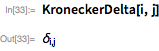The importance of KroneckerDelta lies in the fact that it allows us to “sift” a tensor and isolate individual terms from it with ease. In order to understand this idea, let us obtain the definition of this tensor by applying PiecewiseExpand to it.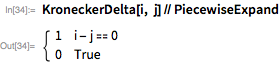From the above, we see that KroneckerDelta[i, j] is 1 if its components i and j are equal, and is equal to 0 otherwise. As a result, it allows us to sift through all the terms in the following sum and select, say, the third term f(3) from it.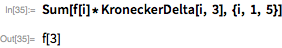In Version 11.1, D makes use of this property of KroneckerDelta to differentiate finite sums with symbolic upper limits with respect to an indexed variable x(j), as illustrated here.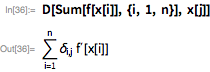The last result expresses the fact that only the jth term in the derivative is nonzero, since none of the other terms depend on x(j), and hence their derivatives with respect to this variable are 0. For example, if we set n=5 and j=2, then the sum reduces to the single term f(x(2)).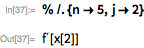Along with the improvements for the functionality of D, Version 11.1 also includes a major documentation update for this important function. In particular, the reference page now includes many application examples of the types encountered in a typical college calculus course. These examples are based on a large collection of more than 5,000 textbook exercises that were solved by a group of talented interns using the Wolfram Language during the summer of 2016. Some of the graphics from these examples are shown here. You can click anywhere inside each of the three following graphics to view their corresponding examples in the online documentation.

D is a venerable function that has been available since Version 1.0 (1988). We hope that the enhancements for this function in Version 11.1 will make it even more appealing to users at all levels. Any comments or feedback about the new features are very welcome.